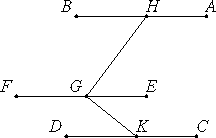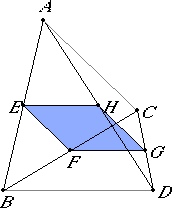# Proposition 9

Straight lines which are parallel to the same straight line but do not lie in the same plane with it are also parallel to each other.

Let each of the straight lines AB and CD be parallel to EF, but not in the same plane with it.

I say that AB is parallel to CD.I.11

Let a point G be taken at random on EF, and from it draw GH in the plane through EF and AB at right angles to EF, and GK in the plane through EF and CD again at right angles to EF.

XI.4

Now, since EF is at right angles to each of the straight lines GH and GK, therefore EF is also at right angles to the plane through GH and GK.

XI.8

And EF is parallel to AB, therefore AB is also at right angles to the plane through HG and GK.

For the same reason CD is also at right angles to the plane through HG and GK. Therefore each of the straight lines AB and CD is at right angles to the plane through HG and GK.

XI.6

But if two straight lines are at right angles to the same plane, then the straight lines are parallel. Therefore AB is parallel to CD.

Therefore, straight lines which are parallel to the same straight line but do not lie in the same plane with it are also parallel to each other.

Q.E.D.

## Guide

Note that this proposition is the three-dimensional analogue to proposition I.30.

#### The Varignon parallelogram of space quadrilaterals

 Consider a quadrilateral ABCD whose four vertices may or may not lie in a plane. Let E, F, G, and H be the midpoints of the sides AB, BC, CD, and DA, respectively. Then the quadrilateral EFGH lies in a plane and is a parallelogram, called the Varignon parallelogram. Varignon (1654–1722) showed the area of a planar quadrilateral is twice the area of this parallelogram. The proof that EFGH is a parallelogram relies on this proposition XI.9 to show the sides are parallel, since it is readily shown that both EF and HG are parallel to the line AC, and both FG and EH are parallel to the line BD. As a corollary, it follows that the lines joining the midpoints of an arbitrary quadrilateral are concurrent and bisect each other, even if the four sides of the quadrilateral do not lie in a plane. (These are the lines EG and FH which are not drawn in the diagram.)#### Use of this proposition

This proposition is used in the proof of the next proposition as well as others in this and the next book.